SBOA345 June   2021

# 4 Calculating Voltage Noise at the Output

Now, we run through a hand calculation to find the total noise of a simplified audio system. For this example, we are using the OPA1656 in a non-inverting configuration with a gain of 40 dB or 100V/V. We are also using filtering as described in Section 1 to limit the BW. Figure 4-1 is the circuit configuration we are using for this example.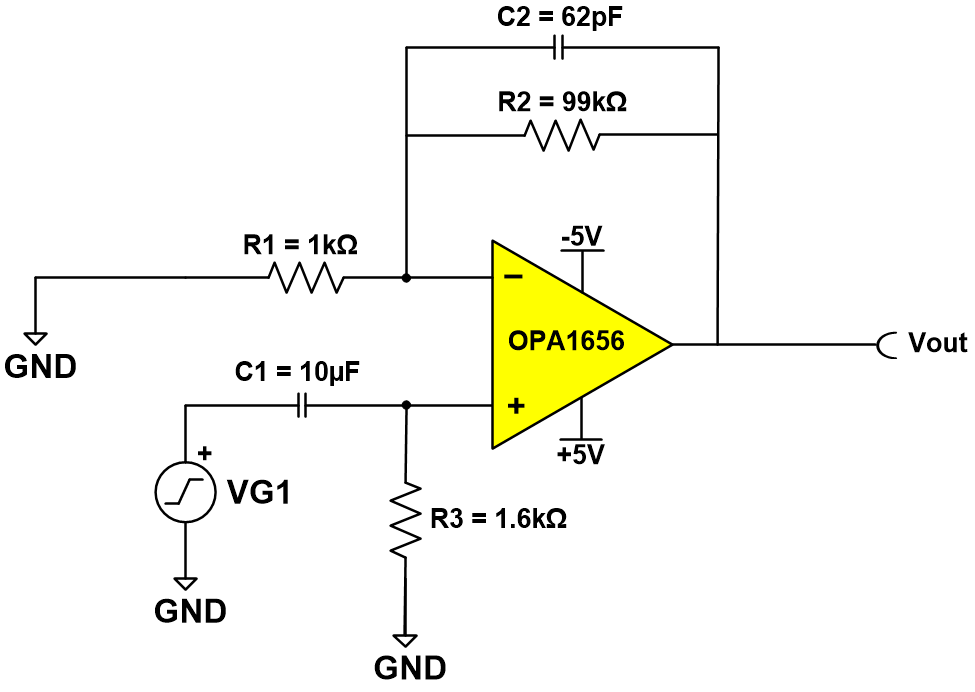Figure 4-1 Circuit Configuration

The first step is to calculate the noise BW of the circuit. The cutoff frequency of the circuit in the example is set at 25.9K. The bandwidth noise of our circuit can be calculated using Equation 5.

Equation 5.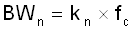Where:

• BWn = Noise BW
• Kn – Brick wall factor (1.57 for a first order filter)
• Fc = Cutoff frequency

Equation 6 is the noise bandwidth for the circuit shown in Figure 4-1.

Equation 6.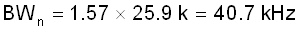We start by looking at the thermal noise for the circuit. The thermal noise a circuit that can be calculated by first calculating the Req of the gain network. This is completed by putting R1 and R2 in parallel. For the circuit shown in Circuit 1 this is done using the following:

Equation 7.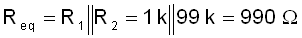At higher speeds the DC blocking cap will act like a short. For this reason, the R4 resistor is considered in parallel with the source resistance. This value is very low and for that reason is not included in the Req calculation. Using Equation 1, the 2RMS voltage noise contribution from the resistors can be calculated.

Equation 8.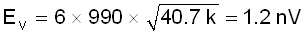Next, we look at the voltage noise of the circuit. When evaluating the voltage noise contribution from the operational amplifier, the first step is to take the input voltage noise shown in the data sheet and multiply it by the square root of the frequency. If we look at the voltage noise from the OPA1656 SoundPlus Ultra-low Noise and Distortion, Burr-Brown Audio Operational Amplifier data sheet we see that the value is 2.9 nV/rtHz at 10kHz. As we have shown in Figure 1-1 the broadband noise will remain flat across frequency, so we can use this number for any frequencies past 10KHz. Taking the voltage noise of the amplifier times our cutoff frequency will yield the RMS voltage noise of the amplifier.

Equation 9.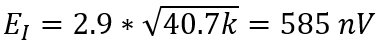The next step is to calculate the current noise contribution of the amplifier. Using Equation 4 it is possible to calculate the RMS current noise contribution of the amplifier. For this example, the current noise of the OPA1656 is 6 fA/rtHz.

Equation 10.Next is the calculation for the total noise at the input of the amplifier.
Equation 11.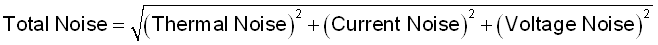Equation 12.This will give what is known as the total input refereed voltage noise. Next multiply the input referred voltage noise by the voltage noise gain, which for our circuit is 100V/V. Remember this is the gain at the non-inverting terminal of the amplifier.

Equation 13.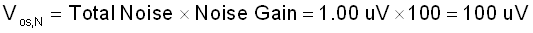Based on our calculations we have a value of 100uVpp. This is very close to the simulated value of the circuit shown in Figure 4-2. Note that for a more accurate calculation, the 1/f noise may be calculated separately. For more information on voltage noise and how to calculate this error, follow these training videos: TI Precision Labs - Op Amps: Noise.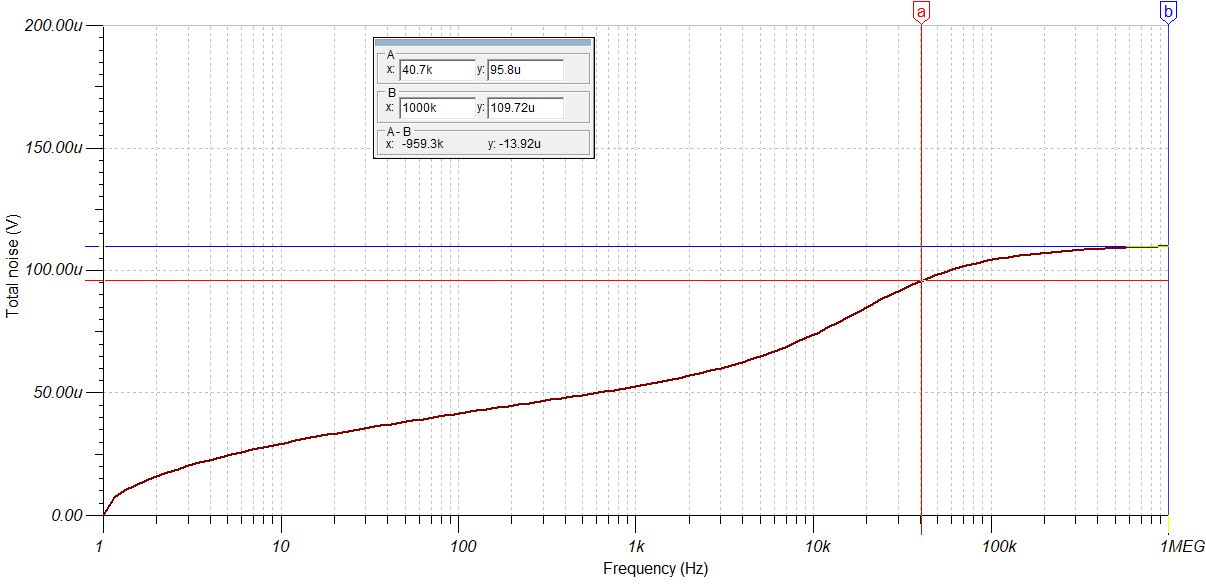Figure 4-2 Simulation Circuit

One of the key reasons for doing the calculations manually, is that it allows you to evaluate the individual noise sources. In our example, we actually see that the resistor noise is our highest noise source.